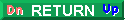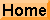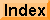10-17 meters# More at 10 attometers

### Strings and Supersymmetry

There is some pretty heady stuff going on in physics these days. In universities around the world, scores of physicists and mathematicians are working on unifying the large and the small into one grand, all-encompassing, theory of everything. The concept is called string theory.

For the past 70 years or so, two theories have dominated physics -- general relativity and quantum mechanics. They have dominated because they work so well at describing the universe as it is. Both make predictions about the universe that have proved to be true as accurately as anyone can measure. General relativity describes the universe at the largest scales. Quantum mechanics describes the universe at the smallest scales. However, there is one inescapable reality: The two theories are mutually incompatible and cannot both be true as currently formulated.

For most objects in the universe, one can accurately use one theory while ignoring the effects of the other -- until one considers objects that combine the extremes of tremendous mass compressed into incredibly small volume. For black holes and the big bang, both quantum mechanics and general relativity apply -- and the answers don't make sense.

### Enter string theory

The theory and equations of general relativity assume that particles can be considered true points -- that there is no limit to the minimum size of matter (electrons, quarks) or force (photons, gluons) particles. This assumption creates problems when attempting to solve problems involving large mass in a small volume. One gets infinite densities, infinite pressures, and infinite temperatures. Essentially the equations return answers that can't be interpreted.

String theory puts a lower limit on the size of particles. In string theory, particles are one dimensional, vibrating strings with a minimum size of a planck length, or 10-35 meters. When considered in this way, the combined equations from quantum mechanics and general relativity produce answers that have changed the way physicists think about the universe. Kevin Green has written an excellent book on string theory called The Theory of Strings.Copyright © 2016 by Bruce Bryson